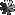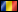User Pass 2FAAuthor Message810

[Mentally Stable]Status: Offline
(since 06-10-2014 15:24)
Joined: 05 Oct 2014
Posts: 1, Topics: 1
Location:Romania

Reputation: 32Imi rezolva cineva urmatorul exercitiu ? : a+b = 12 ab=24 Sa se calculeze : A la a 2 a + B la a 2 a A la a 3 a + B la a 3 a A la a 4 a + B la a 4 a A la a 6 a + B la a 6 a Si : 1 supra A + 1 supra B supra - impartit Si 1 supra radical din 3 totul la -3 este egal cu ?00[Nutella Lover]Status: Offline
(since 13-12-2019 12:16)
Joined: 12 Dec 2012
Posts: 1046, Topics: 193
Location:Gotham

Reputation: 393.9a+b = 12 ab=24 Sa se calculeze : A la a 2 a + B la a 2 a =(a+b)^2-2ab=144-48=96 A la a 3 a + B la a 3 a =(a+b)*(a^2-ab+b^2)=12*84=1008 A la a 4 a + B la a 4 a =(a^2+b^2)^2-2a^2b^2=96^2-2*24^2=8064 A la a 6 a + B la a 6 a =(a^3+b^3)^2-2a^3b^3=1008^2-2*24^3=988416 Si : 1 supra A + 1 supra B =(a+b)/(a*b)=1/2 supra - impartit Si 1 supra radical din 3 totul la -3 =sqrt(3)^3=3sqrt(3) ...sper sa intelegi ce-am scris pe-acolo00Topic lockedThe time now is 21-01-2020, 12:47:03
 Copyright info Based on phpBB ro/comThis message appears only once, so
like us now until it's too late ! :D
x